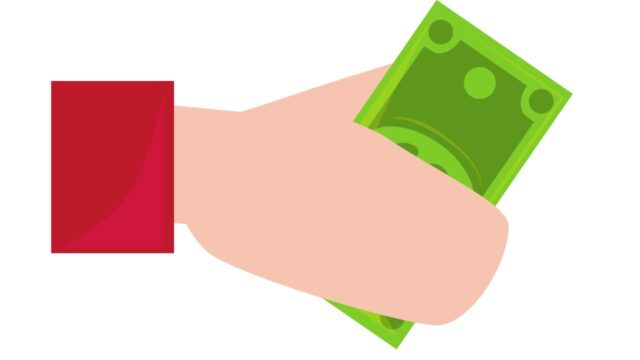# Salvage Value## What is a ‘Salvage Value’

Salvage value is the estimated value that the owner is paid when the item is sold at the end of its useful life. The value is used to determine annual depreciation in the accounting records, and salvage value is used to calculate depreciation expense on the tax return. The value is based on an estimate of the asset’s value, or the value can be determined by a regulatory body, such as the U.S. Internal Revenue Service (IRS).

## Explaining ‘Salvage Value’

The salvage value is used in conjunction with the purchase price and a specific accounting method to determine the amount of annual depreciation on the asset. A business owner can choose the straight-line depreciation method, which means that an equal amount of depreciation is recognized each year. If, instead, the owner chooses an accelerated method of depreciation, the company recognizes more depreciation in the early years and less in the later years of the asset’s useful life.

## How Straight-Line Depreciation Works

Assume, for example, that a company buys a machine at a cost of \$5,000, and that the machine has a value of \$1,000 and a useful life of five years. Based on these assumptions, the annual depreciation using the straight-line method is: (\$5,000 cost – \$1,000 salvage value) / 5 years, or \$800 per year. The asset’s depreciable base is the cost less value, or \$4,000. Salvage value is subtracted from the cost of the asset in the depreciation calculation, because the owner sells the asset once the depreciated value declines.

## How to calculate salvage value

It is the estimated amount that an asset is worth at the end of its useful life. To calculate it, you will need to know the estimated length of the asset’s useful life and its expected rate of depreciation. The salvage value is then calculated by subtracting the total depreciation from the original purchase price. For example, if an asset has a useful life of 10 years and is expected to depreciate at a rate of 10% per year, its value would be calculated as follows: Original purchase price – (10 years x 10% depreciation) = Salvage value. In this case, the salvage value would be \$0. So, when calculating salvage value, it is important to keep in mind that it is not always equal to the original purchase price.

## Factors that influence salvage value

There are a number of factors that can influence the salvage value of a piece of equipment or machinery. Age, condition, and brand are all important considerations. Generally speaking, older items will have a lower salvage value than newer ones. Likewise, items that are in poor condition will have a lower salvage value than those that are well-maintained. And finally, some brands are simply more valuable than others. For example, a piece of Caterpillar equipment is likely to have a higher salvage value than a similar piece of equipment from a lesser-known brand. Of course, these are just general guidelines – ultimately, the actual salvage value of an item will be determined by the market conditions at the time it is sold.

## Examples of how to use salvage value

When an asset is sold, its salvage value is the amount that the selling price exceeds the book value. The salvage value is important because it represents the potential profit that can be made from the sale of an asset. For example, if a company sells a piece of equipment for \$1,000 and its book value is \$700, then the salvage value is \$300. The salvage value can also be used to reduce the cost of an asset. For example, if a company purchases a piece of equipment for \$1,000 and its expected salvage value is \$200, then the cost of the asset is \$800. The salvage value can also be used to calculate the depreciation expense. For example, if an asset has a cost of \$1,000 and an expected salvage value of \$200, then the depreciation expense is \$800 per year.

## Factoring in Accelerated Deprecation Methods

Accelerated depreciation means that the asset’s depreciation is greater in the early years of the useful life and a smaller amount in later years. One popular method is the double-declining balance (DDB) method, which uses a depreciation rate that is twice is straight-line percentage. In the machine example, the rate of annual depreciation is (\$800 annual depreciation / \$4,000 depreciable base), or 20%. The DDB method calculates the first year of depreciation on the machine as (\$5,000 machine cost X 40%), of \$2,000. Because DDB uses a rate that is twice as large as the straight-line rate, more depreciation is recognized in the early years of the asset’s useful life.